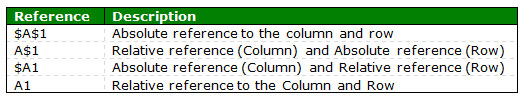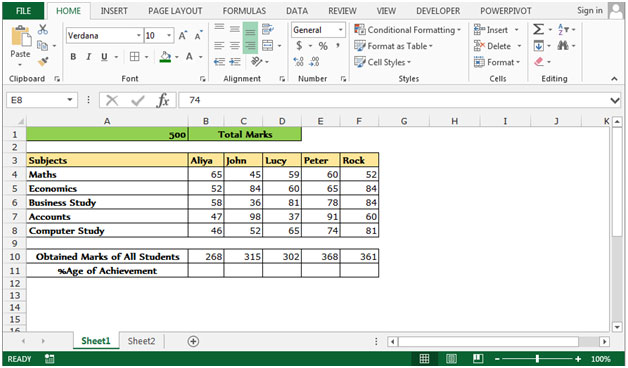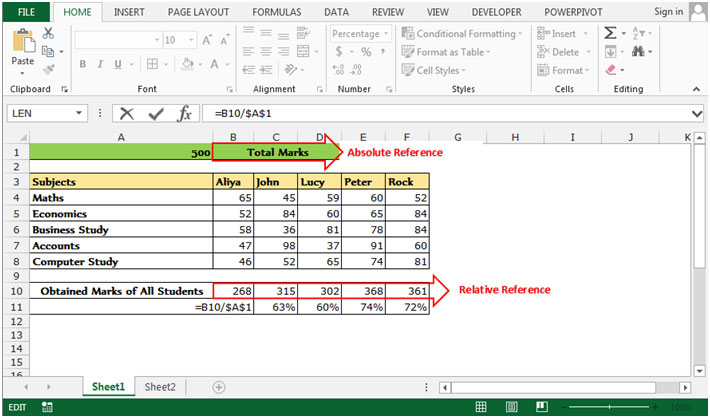# Changing an absolute cell reference to a relative reference in Microsoft Excel

Cell references in Excel are very important. In this article we will learn difference between absolute cell reference & relative cell reference in excel & then learn how to change an absolute reference to a relative reference or vice versa in Microsoft Excel 2010. When a formula is copied, a Relative reference is used. Excel Relative reference is the distance in rows and columns between the reference and the cell that contains the formula.We use the excel absolute reference shortcut F4 key.

Let’s take an example to understand how we can change the relative cell reference from absolute cell reference and  absolute to relative.

We have data in the range A4:F9 in which column A contains subjects and range B4:F9 contains the score of the students. Cell A1 contains the total marks. We need to calculate the percentage of students' scores.In this case, we define the cell A1 as absolute reference.

Absolute reference to the column and row and Relative reference to the Column and Row

• Write the formula for returning the %age of achievement.
• Select the cell B11.
• =B10/\$A\$1 (%age of Achievement = obtained marks/total marks).
• In this example, A1 is our absolute reference for all the students.
• And B11 is our relative reference.
• By pressing the key F4, we make it (A1) absolute reference.
• When we copy the same formula for returning the percentage of all the students, that time all relative reference will get changed but absolute reference (\$A\$1) will never change.This is the way by which we can use the Absolute references and relative references in our formulae by using the key F4 in Microsoft Excel 2010 and 2013.

1.THIS IS THE EXACT THING I WAS LOOKING FOR. THANKS ALOT

2.BAshir Amarkhail

thanks you so much i got what i was looking for from long time

3.Can you discuss how a formula is written using real relative references, such as the R1C1 designations. Using =AVERAGE(M\$90:M\$100) will not calculate the average of the last ten rows if the user inserts new data rows between row 90 and 10>

4.Can you discuss how a formula is written using real relative references, such as the R1C1 designations. Using =AVERAGE(M\$90:M\$100) will not calculate the average of the last ten rows if the user inserts new data rows between row 90 and 10>

Terms and Conditions of use

The applications/code on this site are distributed as is and without warranties or liability. In no event shall the owner of the copyrights, or the authors of the applications/code be liable for any loss of profit, any problems or any damage resulting from the use or evaluation of the applications/code.